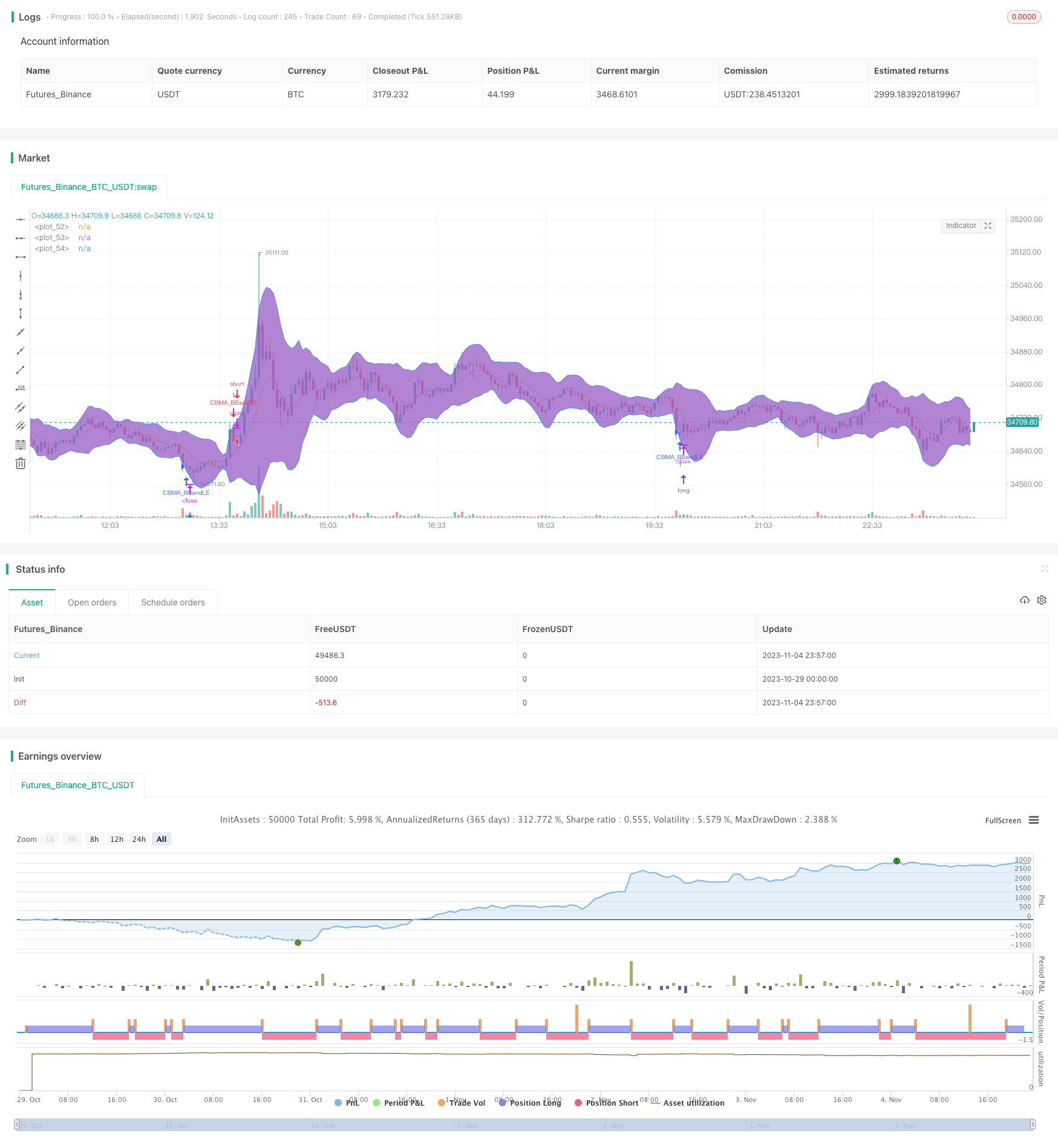# CBMA Bollinger Bands Breaker Strategy

Author: ChaoZhang, Date: 2023-11-06 16:25:01
Tags:## Overview

This strategy uses CBMA as the major technical indicator combined with Bollinger Bands to identify market trends and implement a breaker strategy. It goes short when price breaks above the upper band and goes long when price breaks below the lower band, belonging to the popular dual-track breakout system.

## Strategy Logic

1. Calculate CBMA: Use adaptive EMA to smooth CBMA which can track price changes effectively.

2. Set Bollinger Bands parameters: Use CBMA as middle band, and set upper/lower bands using standard deviation multiplier, which can be adjusted based on market.

3. Breakout trading: Sell when price breaks above upper band, buy when price breaks below lower band, using trend following breaker strategy.

4. Use cancel flash orders, only trade one direction at a time.

5. Set fixed order size, can be adjusted based on capital.

1. CBMA has good smoothness and can track price effectively.

2. Adaptive EMA optimizes the responsiveness of moving average.

3. Upper/lower bands give clear directional signals when breakout happens.

4. Trend following model avoids whipsaw trades.

5. Fixed order size controls single trade risk.

## Risk Analysis

1. Bollinger Bands parameters need optimization, too wide or too narrow can cause issues.

2. Breakout signals may have false breakouts.

3. Need stop loss to control loss.

4. Fixed order size cannot adjust position based on market.

5. Only trade one direction, cannot profit more.

## Optimization Directions

1. Dynamically optimize Bollinger Bands parameters to fit market better.

2. Add more indicators for filtration, avoid false breakouts.

3. Add trailing stop loss to lock in profits.

4. Hedge trading, go both long and short for bigger profit.

## Conclusion

This strategy is a breaker trend following system using adaptive moving average technology combined with Bollinger Bands for clear breakout signals. It has simple logic and fixed order size controls risk, having some practical value. But issues like false breakouts and parameter optimization remain, which need more indicators to improve and enhance real trading performance while controlling risk. Overall it is a decent starter breakout system with much room for improvement.

```/*backtest
start: 2023-10-29 00:00:00
end: 2023-11-05 00:00:00
period: 3m
basePeriod: 1m
exchanges: [{"eid":"Futures_Binance","currency":"BTC_USDT"}]
*/

//@version=4
strategy(title="CBMA Bollinger Bands Strategy directed [ChuckBanger]", shorttitle="CBMA BB CB",
overlay=true )

length = input(title="Length", type=input.integer, defval=12, minval=1)
regular = input(title="Regular BB Or CBMA?", type=input.bool, defval=false)
src = input(title="Source", type=input.source, defval=close)
mult = input(title="Multipler", type=input.float, defval=2.3, minval=.001, maxval=50, step=.1)
emaLen = input(title="EMA Length", type=input.integer, defval=11, minval=1)
emaGL = input(title="EMA Gain Limit", type=input.integer, defval=50, minval=1)
highlight = input(title="Highlight On/Off", type=input.bool, defval=true)

direction = input(0, title = "Strategy Direction", type=input.integer, minval=-1, maxval=1)

strategy.risk.allow_entry_in(direction == 0 ? strategy.direction.all : (direction < 0 ? strategy.direction.short : strategy.direction.long))

//strategy.risk.max_drawdown(50, strategy.percent_of_equity)

calc_hma(src, length) =>
hullma = wma(2*wma(src, length/2)-wma(src, length), round(sqrt(length)))
hullma

calc_cbma(price, length, emaLength, emaGainLimit) =>
alpha = 2 / (emaLength + 1)
ema = ema(price, emaLength)
int leastError = 1000000

float ec = 0
float bestGain = 0

for i = emaGainLimit to emaGainLimit
gain = i / 10
ec := alpha * ( ema + gain * (price - nz(ec)) ) + (1 - alpha) * nz(ec)
error = price - ec
if (abs(error) < leastError)
leastError = abs(error)
bestGain = gain

ec := alpha * ( ema + bestGain * (price - nz(ec)) ) + (1 - alpha) * nz(ec)
hull = calc_hma(price, length)

cbma = (ec + hull) / 2
cbma

cbma = calc_cbma(src, length, emaLen, emaGL)
basis = regular ? sma(src, length) : cbma
dev = mult * stdev(src, length)
upper = basis + dev
lower = basis - dev
cbmaColor = fixnan(highlight and not regular ? cbma > high ? color.purple : cbma < low ? color.aqua : na : color.red)
plot(basis, color=cbmaColor)
p1 = plot(upper, color=color.blue)
p2 = plot(lower, color=color.blue)
fill(p1, p2)

if (crossover(src, lower))
strategy.entry("CBMA_BBandLE", strategy.long, stop=lower, oca_name="BollingerBands", comment="CBMA_BBandLE")
else
strategy.cancel(id="CBMA_BBandLE")

if (crossunder(src, upper))
strategy.entry("CBMA_BBandSE", strategy.short, stop=upper, oca_name="BollingerBands", comment="CBMA_BBandSE")
else
strategy.cancel(id="CBMA_BBandSE")

```

More